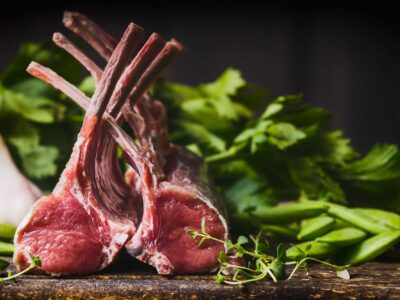Boneless Lamb Leg Roast Lamb\$33 jQuery(function(\$){ var price = '33'; currency = '\$'; /* \$('[name=quantity]').change(function(){ if (!(this.value < 1)) { var product_total = parseFloat(price * this.value); \$(this).closest('.woocom-list-content').find('.product_total_price .price .woocommerce-Price-amount').html(currency + product_total.toFixed(2)); } }); jQuery( '.variations_form' ).each( function() { jQuery(this).on( 'found_variation', function( event, variation ) { var price = variation.display_price; var quantity = \$(this).find('[name=quantity]').val(); if (!(quantity < 1)) { console.log(price); var product_total = parseFloat(price * quantity); \$(this).closest('.woocom-list-content').find('.product_total_price .price .woocommerce-Price-amount').html(currency + product_total.toFixed(2)); } }); });*/ }); 1 KG1.5 KG2 KG Boneless Lamb Leg Roast quantity ADDBoneless Lamb Leg Steak Lamb\$16 jQuery(function(\$){ var price = '16'; currency = '\$'; /* \$('[name=quantity]').change(function(){ if (!(this.value < 1)) { var product_total = parseFloat(price * this.value); \$(this).closest('.woocom-list-content').find('.product_total_price .price .woocommerce-Price-amount').html(currency + product_total.toFixed(2)); } }); jQuery( '.variations_form' ).each( function() { jQuery(this).on( 'found_variation', function( event, variation ) { var price = variation.display_price; var quantity = \$(this).find('[name=quantity]').val(); if (!(quantity < 1)) { console.log(price); var product_total = parseFloat(price * quantity); \$(this).closest('.woocom-list-content').find('.product_total_price .price .woocommerce-Price-amount').html(currency + product_total.toFixed(2)); } }); });*/ }); 500g1 KG Boneless Lamb Leg Steak quantity ADDButterfly Lamb Leg Lamb\$33 jQuery(function(\$){ var price = '33'; currency = '\$'; /* \$('[name=quantity]').change(function(){ if (!(this.value < 1)) { var product_total = parseFloat(price * this.value); \$(this).closest('.woocom-list-content').find('.product_total_price .price .woocommerce-Price-amount').html(currency + product_total.toFixed(2)); } }); jQuery( '.variations_form' ).each( function() { jQuery(this).on( 'found_variation', function( event, variation ) { var price = variation.display_price; var quantity = \$(this).find('[name=quantity]').val(); if (!(quantity < 1)) { console.log(price); var product_total = parseFloat(price * quantity); \$(this).closest('.woocom-list-content').find('.product_total_price .price .woocommerce-Price-amount').html(currency + product_total.toFixed(2)); } }); });*/ }); 1 KG1.5 KG2 KG Butterfly Lamb Leg quantity ADDLamb Bone In Shoulder Roast Lamb\$33 jQuery(function(\$){ var price = '33'; currency = '\$'; /* \$('[name=quantity]').change(function(){ if (!(this.value < 1)) { var product_total = parseFloat(price * this.value); \$(this).closest('.woocom-list-content').find('.product_total_price .price .woocommerce-Price-amount').html(currency + product_total.toFixed(2)); } }); jQuery( '.variations_form' ).each( function() { jQuery(this).on( 'found_variation', function( event, variation ) { var price = variation.display_price; var quantity = \$(this).find('[name=quantity]').val(); if (!(quantity < 1)) { console.log(price); var product_total = parseFloat(price * quantity); \$(this).closest('.woocom-list-content').find('.product_total_price .price .woocommerce-Price-amount').html(currency + product_total.toFixed(2)); } }); });*/ }); 1.5 KG2 KG Lamb Bone In Shoulder Roast quantity ADDLamb Leg Chops Lamb\$12 jQuery(function(\$){ var price = '12'; currency = '\$'; /* \$('[name=quantity]').change(function(){ if (!(this.value < 1)) { var product_total = parseFloat(price * this.value); \$(this).closest('.woocom-list-content').find('.product_total_price .price .woocommerce-Price-amount').html(currency + product_total.toFixed(2)); } }); jQuery( '.variations_form' ).each( function() { jQuery(this).on( 'found_variation', function( event, variation ) { var price = variation.display_price; var quantity = \$(this).find('[name=quantity]').val(); if (!(quantity < 1)) { console.log(price); var product_total = parseFloat(price * quantity); \$(this).closest('.woocom-list-content').find('.product_total_price .price .woocommerce-Price-amount').html(currency + product_total.toFixed(2)); } }); });*/ }); 500g1 KG Lamb Leg Chops quantity ADDLamb Leg Roast- Bone In Lamb\$23 jQuery(function(\$){ var price = '23'; currency = '\$'; /* \$('[name=quantity]').change(function(){ if (!(this.value < 1)) { var product_total = parseFloat(price * this.value); \$(this).closest('.woocom-list-content').find('.product_total_price .price .woocommerce-Price-amount').html(currency + product_total.toFixed(2)); } }); jQuery( '.variations_form' ).each( function() { jQuery(this).on( 'found_variation', function( event, variation ) { var price = variation.display_price; var quantity = \$(this).find('[name=quantity]').val(); if (!(quantity < 1)) { console.log(price); var product_total = parseFloat(price * quantity); \$(this).closest('.woocom-list-content').find('.product_total_price .price .woocommerce-Price-amount').html(currency + product_total.toFixed(2)); } }); });*/ }); 1.5 KG2 KG Lamb Leg Roast- Bone In quantity ADDLamb Loin Chops Lamb\$20 jQuery(function(\$){ var price = '20'; currency = '\$'; /* \$('[name=quantity]').change(function(){ if (!(this.value < 1)) { var product_total = parseFloat(price * this.value); \$(this).closest('.woocom-list-content').find('.product_total_price .price .woocommerce-Price-amount').html(currency + product_total.toFixed(2)); } }); jQuery( '.variations_form' ).each( function() { jQuery(this).on( 'found_variation', function( event, variation ) { var price = variation.display_price; var quantity = \$(this).find('[name=quantity]').val(); if (!(quantity < 1)) { console.log(price); var product_total = parseFloat(price * quantity); \$(this).closest('.woocom-list-content').find('.product_total_price .price .woocommerce-Price-amount').html(currency + product_total.toFixed(2)); } }); });*/ }); 500g1 KG Lamb Loin Chops quantity ADDLamb Rack Lamb\$25 jQuery(function(\$){ var price = '25'; currency = '\$'; /* \$('[name=quantity]').change(function(){ if (!(this.value < 1)) { var product_total = parseFloat(price * this.value); \$(this).closest('.woocom-list-content').find('.product_total_price .price .woocommerce-Price-amount').html(currency + product_total.toFixed(2)); } }); jQuery( '.variations_form' ).each( function() { jQuery(this).on( 'found_variation', function( event, variation ) { var price = variation.display_price; var quantity = \$(this).find('[name=quantity]').val(); if (!(quantity < 1)) { console.log(price); var product_total = parseFloat(price * quantity); \$(this).closest('.woocom-list-content').find('.product_total_price .price .woocommerce-Price-amount').html(currency + product_total.toFixed(2)); } }); });*/ }); Fat OnLean Lamb Rack quantity ADDLamb Shoulder Chops Lamb\$12 jQuery(function(\$){ var price = '12'; currency = '\$'; /* \$('[name=quantity]').change(function(){ if (!(this.value < 1)) { var product_total = parseFloat(price * this.value); \$(this).closest('.woocom-list-content').find('.product_total_price .price .woocommerce-Price-amount').html(currency + product_total.toFixed(2)); } }); jQuery( '.variations_form' ).each( function() { jQuery(this).on( 'found_variation', function( event, variation ) { var price = variation.display_price; var quantity = \$(this).find('[name=quantity]').val(); if (!(quantity < 1)) { console.log(price); var product_total = parseFloat(price * quantity); \$(this).closest('.woocom-list-content').find('.product_total_price .price .woocommerce-Price-amount').html(currency + product_total.toFixed(2)); } }); });*/ }); 500g1 KG Lamb Shoulder Chops quantity ADD